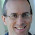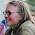## April 24, 2015

### A Radical New Look for Logarithms

"A good notation has a subtlety and suggestiveness which at times make it almost seem like a live teacher." — Bertrand Russell

"We could, of course, use any notation we want; do not laugh at notations; invent them, they are powerful. In fact, mathematics is, to a large extent, invention of better notations." — Richard Feynman

"By relieving the brain of all unnecessary work, a good notation sets it free to concentrate on more advanced problems, and in effect increases the mental power of the race." — Alfred North Whitehead

Notation is perhaps one of the most important aspects of mathematics.  The right choice of notation can make a concept clear as day; the wrong choice can make extracting its meaning hopeless.  Of course, one great thing about notation is that even if there's a poor choice of notation out there (such as $$\left[x\right]$$ or $$\pi$$), often someone comes along and creates a better one (such as $$\lfloor x\rfloor$$ for the floor function or multiples of tau, $$\tau\approx 6.28318$$, for radian measure of angles).

Which brings me to one such poor choice of notation, one that I believe needs fixing:  the rather asymmetrical notation of powers, roots, and logarithms.

Here we have three very closely related concepts — both roots and logarithms are ways to invert exponentiation, the former returning the base and the latter returning the exponent.  And yet their notation couldn't be more different:
$2^3=8\\ \sqrt{8}=2\\ \log_2{8}=3$This always struck me as annoyingly inelegant.  Wouldn't it be nice if these notations bore at least some resemblance to each other?

After giving it some thought, I believe I have found a possible solution.  As an alternative to writing $$\log_2 {8}$$, I propose the following notation:

This notation makes use of a reflected radical symbol, such that the base of the logarithm is written in a similar manner to the index of a radical but below the "point" (the pointy part of the radical symbol), and the argument of the logarithm is written "inside".  The use of this notation has a number of advantages:

1. The symmetry between the normal radical for roots and the reflected radical for logarithms highlights both their similarities and their differences — each one "undoes" an exponential expression, but each one gives a different part of the expression (the base and the exponent, respectively.)
2. The radical symbol can be looked at as a modified lowercase letter "r".  (This may actually be the origin of the symbol, where the "r" stands for radix, the Latin word for "root".)  In a similar way, the new symbol for logarithms resembles a capital "L".
3. The placement of the "small number" and the "point" can take on a secondary spatial meaning:
• The "small number" represents a piece of information we know about an exponential expression, and its placement indicates which part we know.
• For a root, the "small number" is on top, so we know the exponent.
• For a logarithm, the "small number" is on bottom, so we know the base.
• The symbol seems to "point" to the piece of information that we are looking for.
• For a root, the "point" is pointing downward, so we are looking for the base.
• For a logarithm, the "point" is pointing upward, so we are looking for the exponent.
• Looking at the image above, the new notation seems to say "We know the base is 2, so what's the exponent that will get us to 8?"
• Similarly, the expression $$\sqrt{8}$$ now can be interpreted as saying "We know the exponent is 3, so what's the base that will get us to 8?"

This notation would obviously not make much of a difference for seasoned mathematicians who are perfectly comfortable with the $$\log$$ and $$\ln$$ functions.  But from a pedagogical standpoint, the reflected radical, with its multi-layered meaning and auto-mnemonic properties, could help students become more comfortable with a concept that many look at as just meaningless manipulation of symbols.

When I first came up with this reflected-radical notation, I had originally imagined that it should replace the current notation.  However, after some feedback from various people and some further consideration, I think a better course of action would be to have this notation be used alongside the current notation, much in the way that we have multiple notations for other concepts in math (such as the many ways to write derivatives).  However, I would suggest that, if it were to become commonplace*, this notation would be best to use when first introducing the concept in schools.  The current notation isn't wrong per se — it's just not very evocative of the underlying concept.  Anything that can better elucidate that concept can't be a bad thing when it comes to students learning mathematics!

It may seem like a radical idea.
But it's a logical one.

* Of course, for this notation to become commonplace, somebody would need to figure out how to replicate it in LaTeX.  Any takers?

1.am completely on board with this proposal. I never liked log or ln. I always have to remind myself what they mean when I see them.

In light of Shillito's proposal, I think I understand why:

We have this intuitive difference between procedures that are operations and procedures that are functions. Or maybe not even a merely intuitive difference? PEMDAS, after all, is a thing even if the E should really be expanded to include roots and logs.

Yes, we could denote everything with functions. add(3,mul(6,9)) = 57. But we don't, do we? We all know how inefficient that would be, and how much it would obscure the algebraic manipulations we do all the time.

No. We recognize EMDAS, specifically, as operations and assign them unique notational forms which help us to perform the manipulations we need to do.

And note that these operations come in pairs. Plus has minus. Both operations, denoted with symbols that catenate their arguments. Multiplication has division, which likewise have nicely symbolic forms. Exponentiation uses an intuitive placement of arguments to convey its sense, but still, a semantically meaningful way of writing the arguments.

Exponentiation is a little odd, though, in that its two arguments do not have equal relationships to one another. Their roles are fundamentally different. Thus, exponentiation has two inverses: taking roots, and finding logarithms.

Why, then, do roots get an operator representation, while logs are written with what amounts to functional notation? There's not a lot of representational daylight between log_2(x) and sin(x).

So yeah. I'm all for an operator representation for logs, especially one that so nicely mirrors the one for roots and at the same time helps people remember what each one does by literally pointing to the part we're looking for in the corresponding exponential expression.

1."literally pointing to the part we're looking for in the corresponding exponential expression"

Yes, yes, and again yes! This is exactly what I meant. Glad to see that others are understanding why I chose this notation.

If you feel this should be a thing, please share it with whoever you think may be interested!

2.My only problem with the new notation stems from the laziness of the writer. Since 2 is the most natural root and e is the most natural base for logarithms, we don't bother writing the 2 when we take the square root or writing the e when we take logarithm base e (whether you denote this as log or ln is a matter of preference). It wouldn't make sense to compromise on which should be considered natural for both notations since the e^th root doesn't naturally come up and log base 2 doesn't come up much (except maybe in computer applications). I could see how there would be confusion as to why leaving the number off when the sign is in one direction implies something different than leaving the number off when the sign is in the other direction.

That said, I like this idea and think the advantages that you explained outweigh this disadvantage.

1.A computer scientist might argue "2" is the "natural" logarithm base (anything binary).

PS: damn this commenting system is weird.

2.I'm not sure any computer scientist would consider it more natural than e, it's just useful for many purposes.

3.Great idea. I had been searching for a notation that could be "typed" in linear text form. To that end, I had played around with combinations of the caret ^ and forward and reverse diagonals / \

Given the linear notation for power:

Power: b ^ e = p

For root, I suggest the symbol ^/ (caret, slash):

p ^/ e = (b^e) ^/ e = b

My root notation represents an "abbreviation" of the identity: e-th root of p equals p to the power 1/e,
that is: (b^e) ^/e represents (b^e) ^ (1/e) = b ^ (e * 1/e) = b ^ 1 = b

But to get this advantage, I must reverse the order of base and power in the traditional notation.

So, in similar vein, your log symbol could be represented by the symbol: ^\ (caret, back-slash):

log: logb(p) = b ^\ p = b^\ (b^e) = e

This is suggestive of a "visual cancellation rule"
b^\ (b^ e) "equals" ( b^ \ b^) e "equals" 1 * e = e.

1.Interesting idea, but I'm not at all a fan of that "visual cancellation rule" as phrased. There's no real mathematical reason behind it - it's all smoke and mirrors, which is pedagogically dangerous. You're relying on the "b^\b^" resembling a fraction that "cancels" to be 1, after which a multiplication sign magically appears next to the exponent. Math should be logical, not magical. Cancelling things is ubiquitous, sure, but you have to know *why* it works.

That being said, I would be interested in seeing a linear form of the radical symbol and my reflected version of it.

4.Why stopping at the + x exp relation, why not keep going the pattern with the relations due the Conway chained arrow notation? With these simple relations, computation of the V of parallel wires was obtained, it was something nice! Maybe something deeper may happen if the system is generalized.

5.I love your symbol, and will start using it next semester. I made a bit of Latex code for "log base 2 of 8": \raisebox{.7mm}{\reflectbox{\rotatebox[origin=c]{180}{\Large{$\sqrt{~~}$}}}}$\hspace{-.6cm}{\mbox{\tiny 2}}\hspace{.2cm} 8$

To see the compiled image: https://drive.google.com/file/d/1zYqnIFDfsrO-MMQCjI09m_rwmQhqIUfj/view?usp=sharing

6.I'm apparently very late to the party but I absolutely love this idea. I came up with some really nice LaTeX code for it:
\raisebox{\depth}{\scalebox{1}[-1]{$\sqrt[\raisebox{\depth}{\scalebox{1}[-1]{\tiny{2}}}]{\raisebox{\depth}{\scalebox{1}[-1]{16}}}$}}

7.Great write-up, I am a big believer in commenting on blogs to inform the blog writers know that they’ve added something worthwhile to the world wide web!.. correo Hotmail

8.Pretty! This was a really wonderful post. Thank you for providing these details.

www.freesitemaker.net

9.i am browsing this website dailly , and get nice facts from here all the time .

Name

Email *

Message *